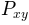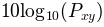Travis-CI:# matplotlib.axes.Axes.csd¶

`Axes.``csd`(x, y, NFFT=None, Fs=None, Fc=None, detrend=None, window=None, noverlap=None, pad_to=None, sides=None, scale_by_freq=None, return_line=None, *, data=None, **kwargs)

Plot the cross-spectral density.

Call signature:

```csd(x, y, NFFT=256, Fs=2, Fc=0, detrend=mlab.detrend_none,
sides='default', scale_by_freq=None, return_line=None, **kwargs)
```

The cross spectral densityby Welch’s average periodogram method. The vectors x and y are divided into NFFT length segments. Each segment is detrended by function detrend and windowed by function window. noverlap gives the length of the overlap between segments. The product of the direct FFTs of x and y are averaged over each segment to compute, with a scaling to correct for power loss due to windowing.

If len(x) < NFFT or len(y) < NFFT, they will be zero padded to NFFT.

Parameters:

x, y : 1-D arrays or sequences

Arrays or sequences containing the data

Fs : scalar

The sampling frequency (samples per time unit). It is used to calculate the Fourier frequencies, freqs, in cycles per time unit. The default value is 2.

window : callable or ndarray

A function or a vector of length NFFT. To create window vectors see `window_hanning()`, `window_none()`, `numpy.blackman()`, `numpy.hamming()`, `numpy.bartlett()`, `scipy.signal()`, `scipy.signal.get_window()`, etc. The default is `window_hanning()`. If a function is passed as the argument, it must take a data segment as an argument and return the windowed version of the segment.

sides : [ ‘default’ | ‘onesided’ | ‘twosided’ ]

Specifies which sides of the spectrum to return. Default gives the default behavior, which returns one-sided for real data and both for complex data. ‘onesided’ forces the return of a one-sided spectrum, while ‘twosided’ forces two-sided.

The number of points to which the data segment is padded when performing the FFT. This can be different from NFFT, which specifies the number of data points used. While not increasing the actual resolution of the spectrum (the minimum distance between resolvable peaks), this can give more points in the plot, allowing for more detail. This corresponds to the n parameter in the call to fft(). The default is None, which sets pad_to equal to NFFT

NFFT : integer

The number of data points used in each block for the FFT. A power 2 is most efficient. The default value is 256. This should NOT be used to get zero padding, or the scaling of the result will be incorrect. Use pad_to for this instead.

detrend : {‘default’, ‘constant’, ‘mean’, ‘linear’, ‘none’} or callable

The function applied to each segment before fft-ing, designed to remove the mean or linear trend. Unlike in MATLAB, where the detrend parameter is a vector, in matplotlib is it a function. The `pylab` module defines `detrend_none()`, `detrend_mean()`, and `detrend_linear()`, but you can use a custom function as well. You can also use a string to choose one of the functions. ‘default’, ‘constant’, and ‘mean’ call `detrend_mean()`. ‘linear’ calls `detrend_linear()`. ‘none’ calls `detrend_none()`.

scale_by_freq : boolean, optional

Specifies whether the resulting density values should be scaled by the scaling frequency, which gives density in units of Hz^-1. This allows for integration over the returned frequency values. The default is True for MATLAB compatibility.

noverlap : integer

The number of points of overlap between segments. The default value is 0 (no overlap).

Fc : integer

The center frequency of x (defaults to 0), which offsets the x extents of the plot to reflect the frequency range used when a signal is acquired and then filtered and downsampled to baseband.

return_line : bool

Whether to include the line object plotted in the returned values. Default is False.

Returns:

Pxy : 1-D array

The values for the cross spectrum `P_{xy}` before scaling (complex valued)

freqs : 1-D array

The frequencies corresponding to the elements in Pxy

line : a `Line2D` instance

The line created by this function. Only returned if return_line is True.

Other Parameters:

**kwargs :

Keyword arguments control the `Line2D` properties:

Property Description
`agg_filter` unknown
`alpha` float (0.0 transparent through 1.0 opaque)
`animated` [True | False]
`antialiased` or aa [True | False]
`clip_box` a `matplotlib.transforms.Bbox` instance
`clip_on` [True | False]
`clip_path` [ (`Path`, `Transform`) | `Patch` | None ]
`color` or c any matplotlib color
`contains` a callable function
`dash_capstyle` [‘butt’ | ‘round’ | ‘projecting’]
`dash_joinstyle` [‘miter’ | ‘round’ | ‘bevel’]
`dashes` sequence of on/off ink in points
`drawstyle` [‘default’ | ‘steps’ | ‘steps-pre’ | ‘steps-mid’ | ‘steps-post’]
`figure` a `matplotlib.figure.Figure` instance
`fillstyle` [‘full’ | ‘left’ | ‘right’ | ‘bottom’ | ‘top’ | ‘none’]
`gid` an id string
`label` string or anything printable with ‘%s’ conversion.
`linestyle` or ls [‘solid’ | ‘dashed’, ‘dashdot’, ‘dotted’ | (offset, on-off-dash-seq) | `'-'` | `'--'` | `'-.'` | `':'` | `'None'` | `' '` | `''`]
`linewidth` or lw float value in points
`marker` `A valid marker style`
`markeredgecolor` or mec any matplotlib color
`markeredgewidth` or mew float value in points
`markerfacecolor` or mfc any matplotlib color
`markerfacecoloralt` or mfcalt any matplotlib color
`markersize` or ms float
`markevery` [None | int | length-2 tuple of int | slice | list/array of int | float | length-2 tuple of float]
`path_effects` unknown
`picker` float distance in points or callable pick function `fn(artist, event)`
`pickradius` float distance in points
`rasterized` [True | False | None]
`sketch_params` unknown
`snap` unknown
`solid_capstyle` [‘butt’ | ‘round’ | ‘projecting’]
`solid_joinstyle` [‘miter’ | ‘round’ | ‘bevel’]
`transform` a `matplotlib.transforms.Transform` instance
`url` a url string
`visible` [True | False]
`xdata` 1D array
`ydata` 1D array
`zorder` any number

`psd()`
`psd()` is the equivalent to setting y=x.
For plotting, the power is plotted asfor decibels, though `P_{xy}` itself is returned.